# Sensitivity analysis using Sobol indices¶

This method deals with analyzing the influence the random vector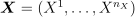has on a random variable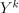which is being studied for uncertainty. Here we attempt to evaluate the part of variance ofdue to the different components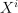.

The estimators for the mean of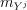and the standard deviationofcan be obtained from a first sample, as Sobol indices estimation requires two samples of the input variables :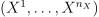, that is two sets of N vectors of dimension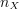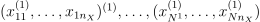and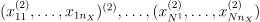The estimation of sensitivity indices for first order consists in estimating the quantity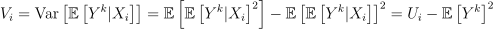Sobol proposes to estimate the quantity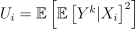by swapping every variables in the two samples except the variable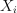between the two calls of the function: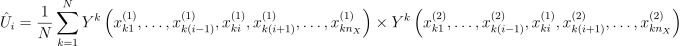Then thefirst order indices are estimated by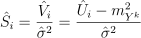For the second order, the two variablesand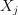are not swapped to estimate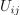, and so on for higher orders, assuming that order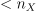. Then the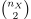second order indices are estimated by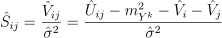For thetotal order indices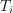, we only swap the variablebetween the two samples.